# Solving Triangles by Reflection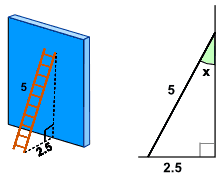A 5ft ladder leans against a wall as shown. What is the angle between the ladder and the wall?

This is surprisingly easy to solve by using Reflection:

 Here is the triangle with its reflection Together they make an equilateral triangle (all sides equal).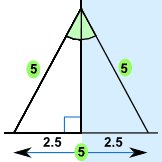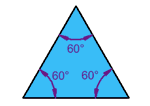The angles in an equilateral triangle are all 60°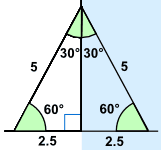So the angle between the ladder and the wall is half of 60° = 30°

## Finding Length

We can use the same idea to find an unknown length.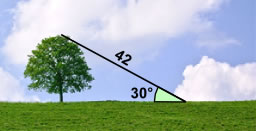Alex has a laser that measures distance.

By standing some distance from the tree Alex measures 42m to the top of the tree at an angle of 30°.

What is the height of the tree?

Here is the triangle and its reflection: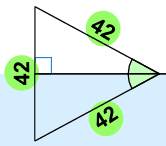Once again the triangle and its reflection make an equilateral triangle. So, we know the height of the tree must be half of 42m = 21m

These examples show that the same triangle can occur in many different situations!